Saturday, July 11, 2020
Home > CBSE Class 12 > NCERT Solutions for Class 12 Maths Vector Algebra Exercise 10.2

# NCERT Solutions for Class 12 Maths Vector Algebra Exercise 10.2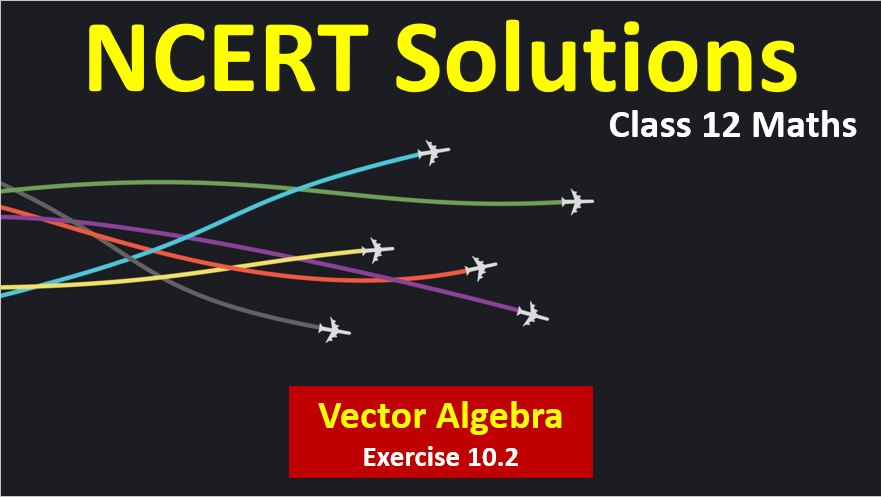# NCERT Solutions for Class 12 Maths Vector Algebra

Hi Students, Welcome to Amans Maths Blogs (AMB). In this post, you will get the NCERT Solutions for Class 12 Maths Vector Algebra Exercise 10.2.

As we know that all the schools affiliated from CBSE follow the NCERT books for all subjects. You can check the CBSE NCERT Syllabus. Thus, NCERT Solutions helps the students to solve the exercise questions as given in NCERT Books.

NCERT Solutions for class 12 is highly recommended by the experienced teacher for students who are going to appear in CBSE Class 12 and JEE Mains and Advanced and NEET level exams. Here You will get NCERT Solutions for Class 12 Maths Vector Algebra Exercise 10.2 of all questions given in NCERT textbooks of class 12 in details with step by step process.

The PDF books of NCERT Solutions for Class 12 are the first step towards the learning and understanding the each sections of Maths, Physics, Chemistry, Biology as it all help in engineering medical entrance exams. To solve it, you just need to click on download links of NCERT solutions for class 12.

CBSE Class 12th is an important school class in your life as you take some serious decision about your career. And out of all subjects, Maths is an important and core subjects. So CBSE NCERT Solutions for Class 12th Maths is major role in your exam preparation as it has detailed chapter wise solutions for all exercise. This NCERT Solutions can be downloaded in PDF file. The downloading link is given at last.

NCERT Solutions for Class 12 Maths are not only the solutions of Maths exercise but it builds your foundation of other important subjects. Getting knowledge of depth concept of CBSE Class 12th Maths like Algebra, Calculus, Trigonometry, Coordinate Geometry help you to understand the concept of Physics and Physical Chemistry.

Note: In this solution, the vector is represented by BOLD font. For example: a, b, OP, AB represent the vectors.

## NCERT Solutions for Class 12 Maths Vector Algebra

NCERT Solutions for Class 12 Maths Vector Algebra Exercise 10.2: Ques No 1.

Compute the magnitude of the following vectors: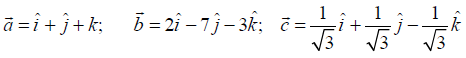NCERT Solutions: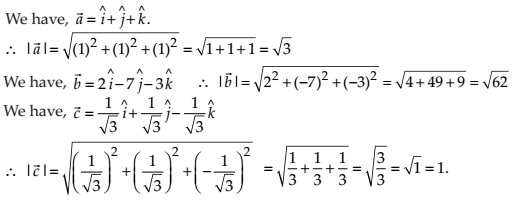NCERT Solutions for Class 12 Maths Vector Algebra Exercise 10.2: Ques No 2.

Write two different vectors having same magnitude.

NCERT Solutions:NCERT Solutions for Class 12 Maths Vector Algebra Exercise 10.2: Ques No 3.

Write two different vectors having same direction.

NCERT Solutions: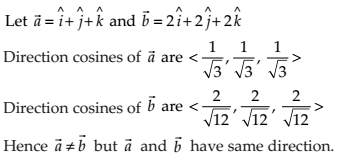NCERT Solutions for Class 12 Maths Vector Algebra Exercise 10.2: Ques No 4.

Find the values of x and y so that the vectors 2i^ + 3j^ and xi^ + yj^ are equal.

NCERT Solutions:

Given that 2i^ + 3j^ and xi^ + yj^ are equal.

Equating the coefficients of i^ and j^, we get x = 2 and y = 3.

NCERT Solutions for Class 12 Maths Vector Algebra Exercise 10.2: Ques No 5.

Find the scalar and vector components of the vector with initial point (2, 1) and terminal point (– 5, 7).

NCERT Solutions:

Let the initial point is A(2, 1) and the terminal point is B(–5, 7).

Thus, the positional vectors areand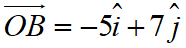Now,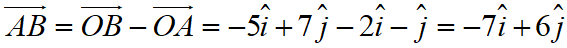Thus, the scalar component of vectors AB is –7 and 6 and vectors component of vectors AB is –7i^ and 6j^.

NCERT Solutions for Class 12 Maths Vector Algebra Exercise 10.2: Ques No 6.

Find the sum of the vectors.

NCERT Solutions:

Given that.

Thus,the sum of the vectors is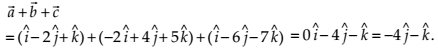NCERT Solutions for Class 12 Maths Vector Algebra Exercise 10.2: Ques No 7.

Find the unit vector in the direction of the vector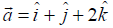.

NCERT Solutions:

Given that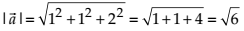Required Unit Vector isNCERT Solutions for Class 12 Maths Vector Algebra Exercise 10.2: Ques No 8.

Find the unit vector in the direction of vectorwhere P and Q are the points (1, 2, 3) and (4, 5, 6), respectively.

NCERT Solutions:

Given that P(1, 2, 3) and Q(4, 5, 6).

Thus,= Positive Vector of Q – Position Vector of P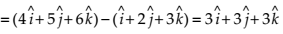Required Unit Vector in the direction of PQ is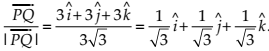NCERT Solutions for Class 12 Maths Vector Algebra Exercise 10.2: Ques No 9.

For the given vectors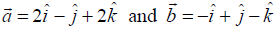, find the unit vector in the direction of the vector.

NCERT Solutions:

Given that.Thus, unit vector in the direction of vectoris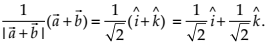NCERT Solutions for Class 12 Maths Vector Algebra Exercise 10.2: Ques No 10.

Find a vector in the direction of vectorwhich has magnitude 8 units.

NCERT Solutions:

Given that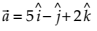.Now, unit vector in the direction of given vector is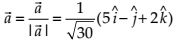Thus, vector of the magnitude of 8 in the direction of vector a is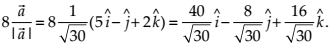NCERT Solutions for Class 12 Maths Vector Algebra Exercise 10.2: Ques No 11.

Show that the vectorsare collinear.

NCERT Solutions:

Given that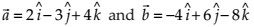.

Now,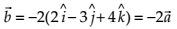The vector b is a scalar multiple of vector a. Thus, the given vectors a and b are collinears.

NCERT Solutions for Class 12 Maths Vector Algebra Exercise 10.2: Ques No 12.

Find the direction cosines of the vector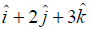.

NCERT Solutions:

Given that.

Thus, the required direction cosine is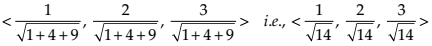NCERT Solutions for Class 12 Maths Vector Algebra Exercise 10.2: Ques No 13.

Find the direction cosines of the vector joining the points A(1, 2, –3) and B(–1, –2, 1), directed from A to B.

NCERT Solutions:

Given that points A(1, 2, –3) and B(–1, –2, 1).

Then, the vector

AB = Position Vector of B – Position Vector of A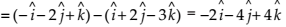.

Thus, the direction cosine of the vector AB is.

NCERT Solutions for Class 12 Maths Vector Algebra Exercise 10.2: Ques No 14.

Show that the vectoris equally inclined to the axes OX, OY and OZ.

NCERT Solutions:

Given that the vector a =.

Then, the direction cosine of the vector a areThus, the given vector is equally inclined to the axes OX, OY and OZ.

NCERT Solutions for Class 12 Maths Vector Algebra Exercise 10.2: Ques No 15.

Find the position vector of a point R which divides the line joining two points P and Q whose position vectors are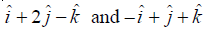respectively, in the ratio 2 : 1.

(i) internally (ii) externally

NCERT Solutions:NCERT Solutions for Class 12 Maths Vector Algebra Exercise 10.2: Ques No 16.

Find the position vector of the mid point of the vector joining the points P(2, 3, 4) and Q(4, 1, –2).

NCERT Solutions: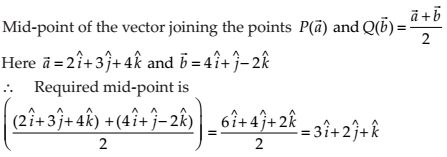NCERT Solutions for Class 12 Maths Vector Algebra Exercise 10.2: Ques No 17.

Show that the points A, B and C with position vectors,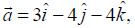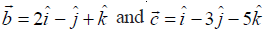and respectively form the vertices of a right angled triangle.

NCERT Solutions:NCERT Solutions for Class 12 Maths Vector Algebra Exercise 10.2: Ques No 18.

In triangle ABC (Fig 10.18), which of the following is not true: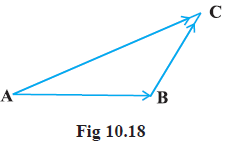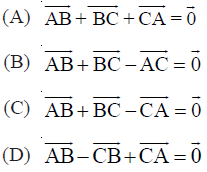NCERT Solutions:

(C) By vectors Law, we have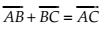.NCERT Solutions for Class 12 Maths Vector Algebra Exercise 10.2: Ques No 19.

If a and b are two collinear vectors, then which of the following are incorrect:

(A) b = λa, for some scalar λ.

(B) a = ±

(C) the respective components of a and b are proportional

(D) both the vectors a and b have same direction, but different magnitudes

NCERT Solutions:

(D) Since a and b are collinear vectors, so it is not necessary that they have same direction.

error: Content is protected !!